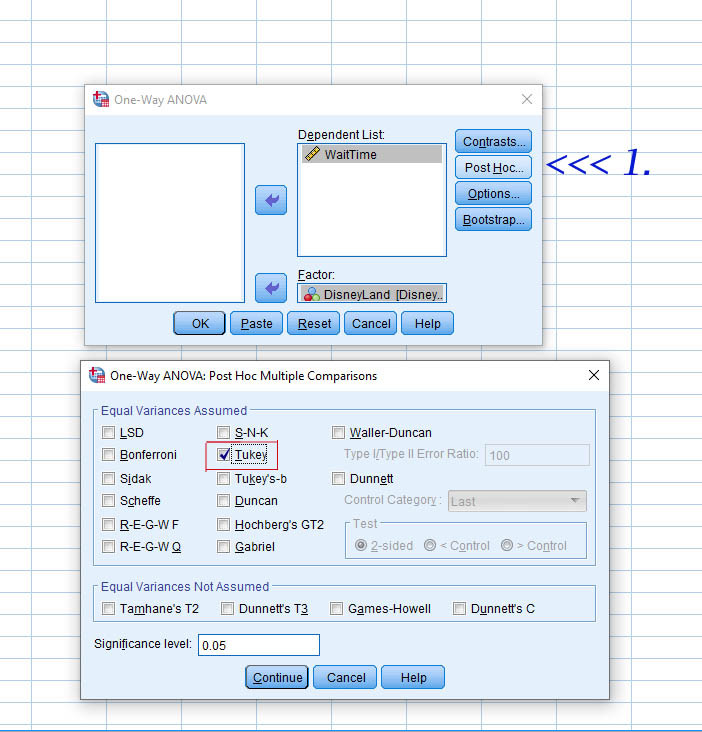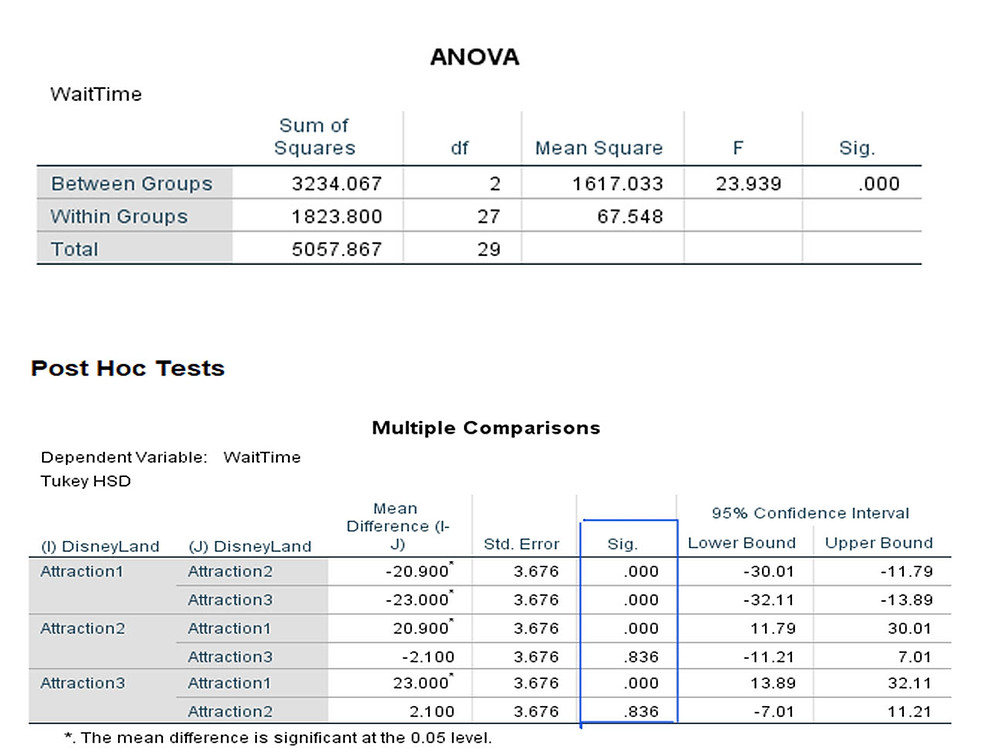Search
• Marek Vavrovic

# One-way ANOVA

The one-way analysis of variance (ANOVA) is used to determine whether there are any statistically significant differences between the means of three or more independent (unrelated) groups.

What does this test do?

The one-way ANOVA compares the means between the groups you are interested in and determines whether any of those means are statistically significantly different from each other. Specifically, it tests the null hypothesis:where µ = group mean and k = number of groups. If, however, the one-way ANOVA returns a statistically significant result, we accept the alternative hypothesis (Ha), which is that there are at least two group means that are statistically significantly different from each other. At this point, it is important to realize that the one-way ANOVA is an omnibus test statistic and cannot tell you which specific groups were statistically significantly different from each other, only that at least two groups were. To determine which specific groups differed from each other, you need to use a post hoc test.

When might you need to use this test?

If you are dealing with individuals, you are likely to encounter this situation using two different types of study design: One study design is to recruit a group of individuals and then randomly split this group into three or more smaller groups (i.e., each participant is allocated to one, and only one, group). You then get each group to undertake different tasks (or put them under different conditions) and measure the outcome/response on the same dependent variable.

Dealing with outliers

Outliers tend to increase the estimate of sample variance, thus decreasing the calculated F statistic for the ANOVA and lowering the chance of rejecting the null hypothesis.

Once a potential outlier has been identified, first check the data to make sure the outlier is not a data entry or data coding error. If not, you can conduct a sensitivity analysis as follows to see how much the outlying observations affect your results.

• Run ANOVA on the entire data.

• Remove outlier(s) and rerun the ANOVA.

• If the results are the same, then you can report the analysis on the full data and report that the outliers did not influence the results.

• If the results are different, try running a non-parametric test (e.g. Kruskal-Wallis) or simply report your analysis with and without the outlier.

For example, if in measuring response times for a rat in maze, suppose the following times were recorded:

20, 21, 24, 26, 30, 31, 33, 95, 230

It is quite possible that two of the rats simply got bored or got distracted and so the results are quite distorted. In this case, the use of a reciprocal transformation tends to reduce the effect of long times. Effectively you are transforming time into speed. The formula for speed is s = d/t, where [s] equals the speed, [d] is the distance covered and [t] is the time it took to cover the distance. The transformed data are:

.0500, .0476, .0417, .0385, .0333, .0323, .0303, .0105, .0043Example 1

We want to find out if the beverage that people dink affects the reaction time. We set up an experiment with 3 groups of people. They are all individual people. We are testing 15 independent samples.

First group gets water to drink, second tea, third coffee. We going to test the reaction time to find out if there is any difference between these groups. The null hypothesis says that the reaction time between all 3 groups is the same. If there were only 2 groups, you can use t-test to find out if there is a difference between them.

When we would do multiple t-test, we are compounding the errors. Each t-test is at α=.05. Compounding multiple t-test gives us significance level of .143 instead of .05.

(.95) (.95) (.95) =.857 α=1-0.857=0.143

The total variation of all the scores is made up of two parts.

-variation within each group. Because the people in each group has different reaction time.

-variation between the groups. Because the drinks you gave them are different.

CONCLUSION: it is the people that make the difference, not the drink.

In this case, you would say that most of the difference is due to the people, and the drink did not make much of a difference. You would accept the null hypothesis that the type of drink does not have any effect on reaction time.Example 2.

Have a look on another example. Now all the scores within each group are very close to each other. There is not a lot of variance within each group, but the groups are quite different from one another. There is a lot of difference between the groups. Conclusion: It is the drink that makes the difference, not the people. In this case you would reject the null hypothesis.Example 3 You are an analyst for Disney Land in China. You have been asked to determine if there is a difference in wait time between the three attractions. To conduct your analysis, you track the wait time for 30 random visitors on each attraction.Transfer the dependent variable Wait Time into the [Dependent List:] box and independent variable Disney Land into the [Factor] box.

Click on the [Post Hoc…] button. Tick the [Turkey ] checkbox as shown below:Click on the [Options…] button. Tick the [Descriptive] checkbox.Anova Table: P-values to too low, means I have to reject the null hypothesis saying all the groups are equal. F- value is too high. F critical = 3.35.

Post Hoc Test: Multiple comparisons.

From the results, we know that there are statistically significant differences between the groups. The table below, Multiple Comparisons, shows which groups differed from each other. The Tukey post hoc test is generally the preferred test for conducting post hoc tests on a one-way ANOVA, but there are many others. We can see from the table below that there is a statistically significant difference in wait time between Attraction1 & 3 and between Attraction1 & 2. However, there were no differences between Attraction2 & 3.## Recent Posts

See All

#### Index

Information functions Filter functions Table manipulation functions Grouping and summarizing Time intelligence functions OPENINGBALANCEYEAR OPENINGBALANCEYEAR(<Expression>,<Dates> [,<Filter>][,<YearEn